JEE  >  JEE Main Previous year questions (2016-20): Thermodynamics

# JEE Main Previous year questions (2016-20): Thermodynamics Notes | Study Chemistry 35 Years JEE Main & Advanced Past year Papers - JEE

## Document Description: JEE Main Previous year questions (2016-20): Thermodynamics for JEE 2022 is part of Thermodynamics for Chemistry 35 Years JEE Main & Advanced Past year Papers preparation. The notes and questions for JEE Main Previous year questions (2016-20): Thermodynamics have been prepared according to the JEE exam syllabus. Information about JEE Main Previous year questions (2016-20): Thermodynamics covers topics like and JEE Main Previous year questions (2016-20): Thermodynamics Example, for JEE 2022 Exam. Find important definitions, questions, notes, meanings, examples, exercises and tests below for JEE Main Previous year questions (2016-20): Thermodynamics.

Introduction of JEE Main Previous year questions (2016-20): Thermodynamics in English is available as part of our Chemistry 35 Years JEE Main & Advanced Past year Papers for JEE & JEE Main Previous year questions (2016-20): Thermodynamics in Hindi for Chemistry 35 Years JEE Main & Advanced Past year Papers course. Download more important topics related with Thermodynamics, notes, lectures and mock test series for JEE Exam by signing up for free. JEE: JEE Main Previous year questions (2016-20): Thermodynamics Notes | Study Chemistry 35 Years JEE Main & Advanced Past year Papers - JEE
 1 Crore+ students have signed up on EduRev. Have you?

Q.1. For the reaction
A(l) → 2B(g)

ΔU = 2.1 kcal, ΔS = 20 cal K−1 at 300 K.
Hence ΔG in kcal is ______.    (2020)
Ans.
(-2.70)
For the reaction
A(l) → 2B(g)
Δng = 2 − 0 = 2
From the relation
ΔH = ΔU + ΔngRT ...(1)
Substituting the values in Eq. (1), we get
ΔH = 2.1 × 10-3 + 2 × 2 × 300 = 3300 cal
We know
ΔG = ΔH − T ΔS ...(2)
Substituting the values in Eq. (2), we get
ΔG = 3300 - 300 × 20
= -2700 cal = - 2.7 kcal

Q.2. The standard heat of formation (ΔfH0298) of ethane (in kJ mol–1), if the heat of combustion of ethane, hydrogen and graphite are −1560, −393.5 and −286 kJ mol–1, respectively is ______.    (2020)
Ans.
(192.5)
We know,
Standard heat of formation of ethane is ΔfH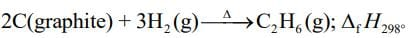Given: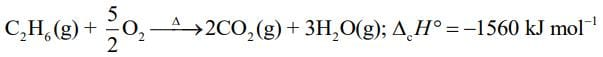...(1)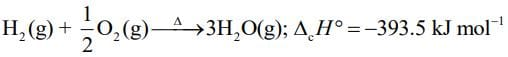...(2)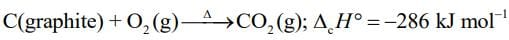...(3)
On applying algebraic operations on equation, that is, 2 × Eq. (3) + 3 × Eq. (2) – Eq. (1), We get,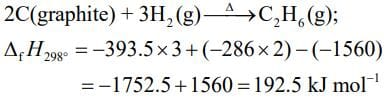Q.3. The magnitude of work done by a gas that undergoes a reversible expansion along the path ABC shown in the figure is ________.    (2020)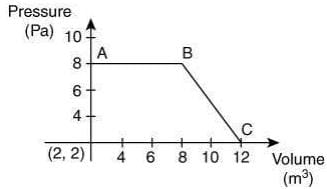Ans.
(4)
The magnitude of word done undergo a reversible expansion along the path ABC is the are of the trapezium ABC.
Thus, the word done is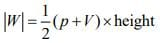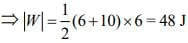Q.4. At constant volume, 4 mol of an ideal gas when heated from 300 K to 500 K changes its internal energy by 5000 J. The molar heat capacity at constant volume is _____.    (2020)
Ans.
(6.25)
We know
ΔU = nCV,mΔT ...(1)
Substituting the given values in Eq. (1), we get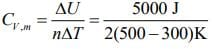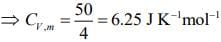Q.5. If enthalpy of atomization for Br2(l) is x kJ mol–1 and bond enthalpy for Br2 is y kJ mol–1, the relation between them    (2020)
(1) is x = y
(2) Does not exist
(3)  is x > y
(4) is x < y
Ans.
(3)
Given, enthalpy of atomization of B2(l) is x kJ mol–1 and bond enthalpy of B2(l) is y kJ mol–1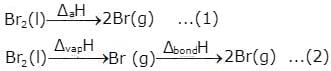From the above two reactions
x = Δvap H + y
⇒ x > y.

Q.6. The true statement amongst the following is    (2020)
(1) Both ΔS and S are functions of temperature.
(2) Both S and ΔS are not functions of temperature.
(3) S is not a function of temperature but ΔS is a function of temperature.
(4) S is a function of temperature but ΔS is not a function of temperature.
Ans.
(1)
Both entropy (S) and change in entropy (ΔS) is the function of temperature.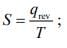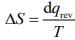Q.7. Consider the reversible isothermal expansion of an ideal gas in a closed system at two different temperatures T1 and T2 (T1 < T2). The correct graphical depiction of the dependence of work done (w) on the final volume (V) is:    (2019)
(1)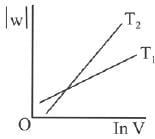(2)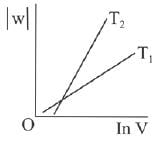(3)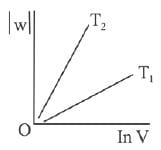(4) |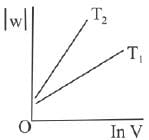Ans.
(2)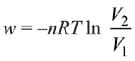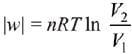|w| = nRT (InV2 - InV1)
|w| = nRT In V2 - nRT V1
y = mx + c
So, slope of curve 2 is more than curve 1 and intercept of curve 2 is more negative than curve 1.

Q.8. The entropy change associated with the conversion of 1 kg of ice at 273 K to water vapours at 383 K is: (Specific heat of water liquid and water vapour are 4.2 kJ K-1 kg-1 and 2.0 kJ K-1 kg-1; heat of liquid fusion and vapourisation of water are 334 kJ kg-1 and 2491 kJ kg-1, respectively).
(log 273 = 2.436, log 373 = 2.572, log 383 = 2.583)    (2019)
(1) 7.90 kJ kg-1 K-1
(2) 2.64 kJ kg-1 K-1
(3) 8.49 kJ kg-1 K-1
(4) 9.26 kJ kg-1 K-1
Ans.
(4)
As we know,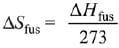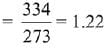Also,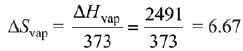Also,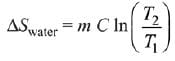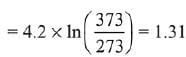Now,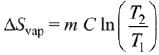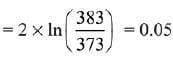∴ Total entropy change
ΔS = 9.26 kJ kg-1 K-1

Q.9. A process has ΔH = 200 J mol-1 and ΔS = 40 JK-1 mol-1. Out of the values given below, choose the minimum temperature above which the process will be spontaneous:    (2019)
(1) 20 K
(2) 12 K
(3) 5 K
(4) 4 K
Ans.
(3)
ΔH = 200 J mol-1
ΔS = 40 JK-1 mol-1
For spontaneous reaction,
ΔG < 0
ΔH - TΔS < 0; ΔH < TΔS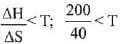5 < T
So, minimum temperature is 5 K.

Q.10. The process with negative entropy change is:    (2019)
(1) Dissociation of CaSO4(s) to CaO(s) and SO3(g)
(2) Sublimation of dry ice
(3) Dissolution of iodine in water
(4) Synthesis of ammonia from N2 and H2
Ans.
(4)
In the process of synthesis of ammonia from N2 and H2, number of moles decreases which implies that the change in entropy will be negative.
N2(g) + 3H2(g) → 2NH3(g)

Q.11. An ideal gas undergoes isothermal compression from 5 m3 to 1 m3 against a constant external pressure of 4 Nm-2. Heat released in this process is used to increase the temperature of 1 mole of Al. If molar heat capacity of Al is 24 J mol-1 K-1, the temperature of Al increases by:    (2019)
(1) 3/2 K
(2) 2 K
(3) 2/3 K
(4) 1 K
Ans.
(3)
We know that,
w = -Pext(Vf - Vi)
w = -4 Nm-2(1 - 5)m3
w = 16 Nm ⇒ 16 J
For isothermal compression,
ΔU = q + w
⇒ q = -w = -16 J (∵ ΔU = 0 for isothermal process)
From calorimetry,
Heat given = n C ΔT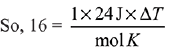∴ Change in temperature, ΔT = 2/3 K

Q.12. Two blocks of the same metal having same mass and at temperature T1, and T2, respectively, are brought in contact with each other and allowed to attain thermal equilibrium at constant pressure. The change in entropy, ΔS, for this process is:    (2019)
(1)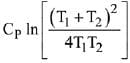(2)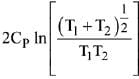(3)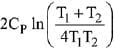(4)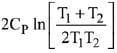Ans. (1)
Final temperature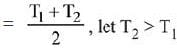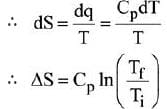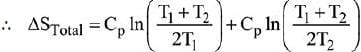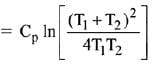Q.13. For the chemical reaction X ⇌ Y, the standard reaction Gibbs energy depends on temperature T (in K) as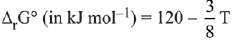The major component of the reaction mixture at T is:    (2019)
(1) Y if T= 300 K
(2) Y if T= 280 K
(3) X if T = 350 K
(4) X if T = 315 K
Ans.
(4)
At 315 K; ΔGº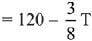ΔG° = 120 - 118.125 = positive
Since ΔG° is positive then Keq < 1.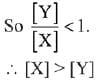Q.14. The standard reaction Gibbs energy for a chemical reaction at an absolute temperature T is given by ΔG° = A - BT where A and B are non-zero constants. Which of the following is true about this reaction?    (2019)
(1) Exothermic if B < 0
(2) Endothermic if A > 0
(3) Endothermic if A < 0 and B > 0
(4) Exothermic if A > 0 and B < 0
Ans.
(3)
MgO (s) + C(s) → Mg (s) + CO (g)
For a reaction to be spontaneous
ΔG < 0
ΔH° - TΔS° < 0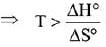T > 2480.3 K

Q.15. For a diatomic ideal gas in a closed system, which of the following plots does not correctly describe the relation between various thermodynamic quantities?    (2019)
(1)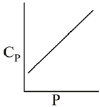(2)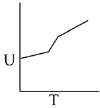(3)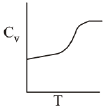(4)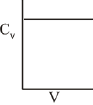Ans.
(2)
ΔG° = A - BT
A and B are non-zero constants
∴ ΔG° = ΔH° - TΔS° = A - BT
∴ Reaction will be endothermic if ΔG° > 0.
Hence, A > O and B < O.

Q.16. Given: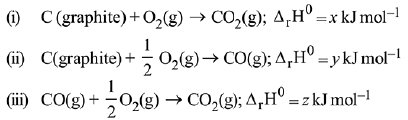Based on the above thermochemical equations, find out which one of the following algebraic relationships is correct?    (2019)
(1) x = y + z
(2) z = x + y
(3) y = 2z - x
(4) x = y - z
Ans.
(1)
(ΔTf)x = (ΔTf)y
Kf.mx = Kf.my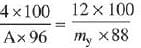my = 3.27 A; y ≈ 3A

Q.17. For silver, Cp(JK-1 mol-1) = 23 + 0.01T. If the temperature (T) of 3 moles of silver is raised from 300 K to 1000 K at 1 atm pressure, the value of ΔH will be close to:    (2019)
(1) 62 kJ
(2) 16 kJ
(3) 21 kJ
(4) 13 kJ
Ans.
(1)
Given: n = 3
T1 = 300; T2 = 1000
Cp =23 + o.01T
The relation between ΔH and C is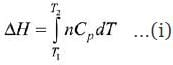After putting all variable values in eq. (i)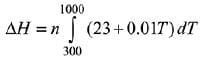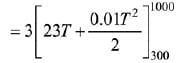= 3[16100 + 4550] = 3 × 20650 = 61950 J
= 61.95 kJ
= 62 kJ

Q.18. Which one of the following equations does not correctly represent the first law of thermodynamics for the given processes involving an ideal gas ? (Assume non-expansion work is zero)    (2019)
(1) Cyclic process : q = -w
(3) Isochoric process: ΔU = q
(4) Isothermal process: q = -w
Ans.
(2)
From first law of thermodynamics, ΔU = q + w
For adiabatic process, q = 0
∴ ΔU = w
For isothermal process, ΔU = 0
For cyclic process, ΔU = 0
For isochoric process, w = 0

Q.19. 5 moles of an ideal gas at 100 K are allowed to undergo reversible compression till its temperature becomes 200 K. If Cv = 28 J K-1 mol-1, calculate ΔU and ΔPV for this process. (R = 8.0 J K-1 mol-1)    (2019)
(1) ΔU = 14 kJ; Δ(PV) = 18 kJ
(2) ΔU = 14 kJ; Δ(PV) = 0.8 kJ
(3) ΔU = 14 kJ; Δ(PV) = 4 kJ
(4) ΔU = 14 kJ; Δ(PV) = 8.0 kJ
Ans.
(3)
ΔU = nCv ΔT = 5 × 28 × 100 = 14 KJ
Δ(PV) = nR (T2 - T1) = 5 × 8 × 100 = 4 KJ

Q.20. Among the following, the set of parameters that represents path functions, is:    (2019)
(a) q + w
(b) q
(c) w
(d) H-TS
(1) (B) and (C)
(2) (B), (C) and (D)
(3)(A) and (D)
(4) (A), (B) and (C)
Ans.
(1)
We know that heat and work are not state functions but q + w = ΔU is a state function. H -TS (i.e. G) is also a state function.

Q.21. Consider the given plot of enthalpy of the following reaction between A and B, A + B → C + D.
Identify the incorrect statement.    (2019)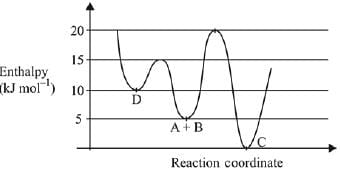(1) Activation enthalpy to form C is 5kJ mol-1 less than that to form D.
(2) C is the thermodynamically stable product.
(3) D is kinetically stable product.
(4) Formation of A and B from C has highest enthalpy of activation.
Ans.
(1)
As we can see from the graph that activation enthalpy to form D from A+B is 15 - 5 = 10 kJ mol-1, whereas, to form C from A + B is 20 - 5 = 15 kJ mol-1. Therefore, activation enthalpy to form C is 5 kJ more than that to form D.

Q.22. During compression of a spring the work done is 10 kJ and 2 kJ escaped to the surroundings as heat. The change in internal energy, ΔU (in kJ) is:    (2019)
(1) -12
(2) -8
(3) 8
(4) 12
Ans.
(3)
w= 10 kJ
q = -2 kJ
ΔU = q + w = -2 + 10 = 8 kJ

Q.23. A process will be spontaneous at all temperatures if:    (2019)
(1) ΔH < 0 and ΔS < 0
(2) ΔH > 0 and ΔS < 0
(3) ΔH < 0 and ΔS > 0
(4) ΔH > 0 and ΔS > 0
Ans.
(3)
A reaction is spontaneous if ΔGsys is negative.
ΔGsys = ΔHsys -TΔSsys
A reaction will be spontaneous at all temperatures if ΔHsys is negative and ΔSsys is positive.

Q.24. The difference between ΔH and ΔU (ΔH-ΔU), when the combustion of one mole of heptane (I) is carried out at a temperature T, is equal to:    (2019)
(1) -4 RT
(2) -3RT
(3) 4RT
(4) 3 RT
Ans.
(1)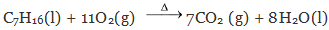ΔH - ΔU = ΔngRT
Δng = no. of moles of product in gaseous state - no. of moles of reactant in gaseous state.
∵ Δng = -4
∴ ΔH - ΔU = -4RT

Q.25. An ideal gas is allowed to expand from 1 L to 10 L against a constant external pressure of 1 bar. The work done in kJ is:    (2019)
(1) -9.0
(2) +10.0
(3) -0.9
(4) -2.0
Ans.
(3)
w = - PΔV
= -(1 bar) x (9 L)
= -(105 Pa) x (9 x 10-3) m3
= - 9 x 102 N.m
= - 900 J
= - 0.9 kJ

Q.26. Enthalpy of sublimation of iodine is 24 cal g-1 at 200°C. If specific heat of I2(s) and I2(vap) are 0.055 and 0.031 cal g-1K-1 respectively, then enthalpy of sublimation of iodine at 250°C in cal g-1 is:    (2019)
(1) 2.85
(2) 5.7
(3) 22.8
(4) 11.4
Ans.
(3)
I2(s) → I2(g).
Heat of reaction depend upon temperature i.e., it varies with temperature, as given by Kirchoff's equation,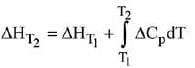where ΔCp = Cp of product - Cp of reactant
∴ ΔCp = 0.031 - 0.055 = - 0.024 cal/g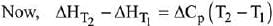ΔH(250) - ΔH(200) = -0.024(523 - 473)
ΔH(250) = 24 - 50 x 0.024 = 22.8 cal/g

Q.27. The INCORRECT match in the following is:    (2019)
(1) ΔG° < 0, K > 1
(2) ΔG° = 0, K = 1
(3) ΔG° > 0, K < 1
(4) ΔG° < 0, K < 1
Ans.
(4)
ΔGº = -RT Ink
∴ If K > 1 then ΔGº < 0
If K < 1 then ΔGº > 0
If K = 1 then ΔGº = 0

Q.28. Which of the following lines correctly show the temperature dependence of equilibrium constant K, for an exothermic reaction?    (2018)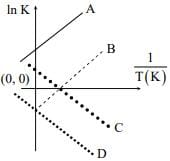(1) A and B
(2) B and C
(3) C and D
(4) A and D
Ans.
(2)
ΔGº = -RT In K
ΔHº - TΔSº = -RT In K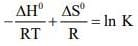Therefore In K vs 1/T graph will be a straight line with slope equal to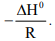Since reaction is exothermic, therefore ΔH0 itself will be negative resulting in positive slope.

Q.29. The combustion of benzene (1) gives CO2(g) and H2O(I). Given that heat of combustion of benzene at constant volume is -3263.9 kJ mol-1 at 250 C; heat of combust ion (in kJ mol-1) of benzene at constant pressure will be:    (2018)
(1) 4152.6
(2) -452.46
(3) 3260
(4) -3267.6

Ans.
(4)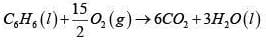Δng = 6-7.5 = -1.5
ΔH  = ΔU + ΔngRT
= -3263.9 - 1.5 x 8.134 x 10-3 x 298
= -3267.6

Q.30. An ideal gas undergoes a cyclic process as shown in Figure.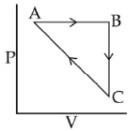ΔUBC = −5 KJ mol−1, qAB = 2KJ mol−1
WAB = −5KJ mol−1, WCA = 3KJ mol−1
Heat absorbed by the system during process CA is:    (2018)
(1) -18 KJ mol-1
(2) -5 kJ mol-1
(3) +5 KJ mol-1
(4) 18 KJ  mol-1
Ans. (3)
AB → isobaric
BC → Isochoric
CA → not defined
ΔUAB = q + W
= 2 − 5 = −3
ΔUABC=ΔUAB+ΔUBC
=− 3 − 5= − 8 kJ
ΔUCBA = +8
= Q + W
8 = Q + 3
Q = +5 kJ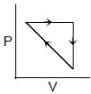Q.31. For which of the following processes, ΔS is negative?    (2018)
(1) C(diamond) → C(graphite)
(2) N2(g, 1 atm) → N2(g, 5 atm)
(3) N2(g, 273 K) → N2(g, 300 K)
(4) H2(g) → 2H(g)
Ans.
(2)
N2 (g, 1 atm) → N2 (g, 5 atm)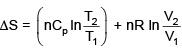for isothermal process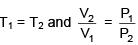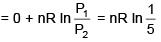Therefore, ΔS < 0

Q.32. ΔU is equal to    (2017)
(1) Isochoric work
(2) Isobaric work
(4) Isothermal work
Ans.
(3)
For adiabatic process, q = 0
∴ As per 1st law of thermodynamics,
ΔU = W

Q.33. For a reaction, A(g) → A(l); ΔH = –3RT.
The correct statement for the reaction is:    (2017)
(1) ΔH = ΔU = 0
(2) |ΔH| < |ΔU|
(3) |ΔH| > |ΔU|
(4) ΔH = ΔU ≠ 0
Ans.
(3)
For the reaction A(g) → A(1)
The change in the number of moles of gaseous species Δn = 0 - 1 = -1
The enthalpy change ΔH = -3RT
ΔH = ΔU + ΔnRT
-3RT = ΔU + (-1)RT
The change in internal energy  ΔU = -2RT
Hence, |ΔH| > |ΔU|

Q.34. The enthalpy change on freezing of 1 mol of water at 5°C to ice at –5°C is:    (2017)
(Given Δfus H = 6 kJ mol-1 at 0ºC,
Cp(H2O, ℓ) = 75.3 J mol-1 K-1,
Cp (H2O, S) = 36.8 J mol-1 K-1)
(1) 6.00 kJ mol-1
(2) 5.81 kJ mol-1
(3) 5.44 kJ mol-1
(4) 6.56 kJ mol-1
Ans.
(4)
In order to calculate the enthalpy change for H2O at 5ºC, we need to calculate the enthalpy change of all the transformation involved in the process.
(a) Energy change of 1 mol, H2O(I), at 5ºC → 1 mol, H2O(I), 0ºC
(b) Energy change of 1 mol, H2O(I), at 0ºC → 1 mol, H2O(s)(ice), 0ºC
(c) Energy change of 1 mol, Ice(s), at 0ºC → 1 mol, Ice(s), -50C
Total ΔH = Cp(H2O(l)]ΔT +ΔH freezing + Cp [H2O (s)] ΔT
= (75.3 J mol-1 K-1)(-5)K + (-6 x 103 J mol-1) + (36.8 J mol-1 K-1) (-5) K
ΔH = -6.56 kJ mol-1 (exothermic process)
So, ΔH = 6.56 kJ

Q.35. A gas undergoes change from state A to state B. In this process, the heat absorbed and work done by the gas is 5 J and 8 J, respectively. Now gas is brought back to A by another process during which 3 J of heat is evolved. In this reverse process of B to A:    (2017)
(1) 6J of the work will be done by the gas
(2) 6J of the work will be done by the surrounding on gas.
(3) 10 J of the work will be done by the surrounding on gas.
(4) 10 J of the work will be done by the gas
Ans.
(2)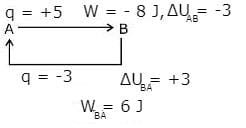Q.36. The heats of combustion of carbon and carbon monoxide are - 393.5 and -283.5 kJ mol-1, respectively.
The heat of formation (in kJ) of carbon monoxide per mole is:    (2016)
(1) 110.5
(2) 676.5
(3) -676.5
(4) -110.5
Ans.
(4)Q.37. A reaction at 1 bar is non-spontaneous at low temperature but becomes spontaneous at high temperature. Identify the correct statement about the reaction among the following:    (2016)
(1) Both ΔH and ΔS are negative.
(2) Both ΔH and ΔS are positive.
(3) ΔH is positive while ΔS is negative.
(4) ΔH is negative while ΔS is positive.
Ans.
(2)
ΔG=ΔH−T. ΔS
If ΔH & ΔS are both positive, then ΔG may be negative at height temperature. Hence reaction becomes spontaneous at higher temperature.

Q.38. If 100 mole of H2O2 decompose at 1 bar and 300 K, the work done (kJ) by one mole of O2(g) as it expands against 1 bar pressure is:
2H2O2(l) ⇌ 2H2O(l) + O2(g)  (R = 8.3 J K–1 mol–1)    (2016)
(1) 498.00
(2) 62.25
(3) 124.50
(4) 249.00
Ans.
(3)
2H2O2(l) ⇌ 2H2O(l) + O2(g)
W = –Pext (ΔV) = –(nO2) RT
∵ 100 mol H2O2 on decomposition will give 50 mol O2
⇒ W = –(50)(8.3)(300)J
= - 124500 J
W = – 124.5 kJ
⇒ Work done by O2(g) = 124.5 kJ

The document JEE Main Previous year questions (2016-20): Thermodynamics Notes | Study Chemistry 35 Years JEE Main & Advanced Past year Papers - JEE is a part of the JEE Course Chemistry 35 Years JEE Main & Advanced Past year Papers.
All you need of JEE at this link: JEE

## Chemistry 35 Years JEE Main & Advanced Past year Papers

122 docs|66 tests
 Use Code STAYHOME200 and get INR 200 additional OFF

## Chemistry 35 Years JEE Main & Advanced Past year Papers

122 docs|66 tests

Track your progress, build streaks, highlight & save important lessons and more!

,

,

,

,

,

,

,

,

,

,

,

,

,

,

,

,

,

,

,

,

,

;